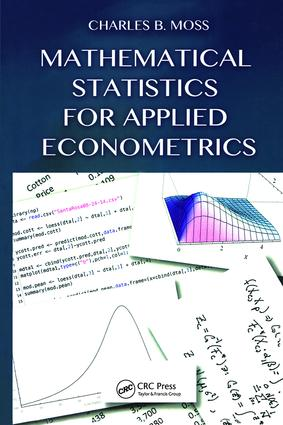# Mathematical Statistics for Applied Econometrics

## 1st Edition

Chapman and Hall/CRC

363 pages | 54 B/W Illus.

##### Purchasing Options:\$ = USD
Hardback: 9781466594098
pub: 2014-10-16
\$115.00
x
eBook (VitalSource) : 9780429168895
pub: 2014-10-16
from \$28.98

FREE Standard Shipping!

### Description

An Introductory Econometrics Text

Mathematical Statistics for Applied Econometrics covers the basics of statistical inference in support of a subsequent course on classical econometrics. The book shows students how mathematical statistics concepts form the basis of econometric formulations. It also helps them think about statistics as more than a toolbox of techniques.

Uses Computer Systems to Simplify Computation

The text explores the unifying themes involved in quantifying sample information to make inferences. After developing the necessary probability theory, it presents the concepts of estimation, such as convergence, point estimators, confidence intervals, and hypothesis tests. The text then shifts from a general development of mathematical statistics to focus on applications particularly popular in economics. It delves into matrix analysis, linear models, and nonlinear econometric techniques.

Students Understand the Reasons for the Results

Avoiding a cookbook approach to econometrics, this textbook develops students’ theoretical understanding of statistical tools and econometric applications. It provides them with the foundation for further econometric studies.

### Reviews

"Its goals are to cover the basics of statistical inference in support of a subsequent econometrics course and to explain the ’why’ to motivate the students who had previously taken an introductory statistics or econometrics course of a cookbook ?avour. Such a textbook is needed, as several popular econometrics textbooks put fundamentals of mathematical statistics in an appendix … [T]he book shows how mathematical statistics is useful in econometrics and economic decisions under uncertainty and risk. It carefully explains the logic underlying estimators and tests, with an emphasis on laying a solid foundation for their uses in the subsequent applications. It is very useful to introduce and use the symbolic programs Maxima and MathematicaTM for the mathematical calculations, in addition to the open source package R for the numerical and graphical tasks. The textbook also has web resources with lecture slides, data sets and computer programs … and can be used both by graduate students from economics, business and various other backgrounds and by instructors and practitioners as a reference."

—Shuangzhe Liu in Stastistical Papers, October 2016

"This book presents a useful and well-integrated connection between mathematical statistics and applied econometrics. There is a natural progression from probability theory to estimations to economics applications, and the examples are helpful to understand and illustrate the statistical concepts. I highly recommend it to students who want to understand statistical theory that is driving econometrics applications frequently used in economics research."

Ani Katchova, Associate Professor, Agricultural Economics, University of Kentucky

"This book is a formal yet accessible introductory textbook to mathematical statistics, with a clear emphasis on building a strong background for the subsequent study of econometric methods employed in social sciences, agricultural economics, finance, and agribusiness. The book’s intended audiences are graduate students—very strong masters and all doctoral students—and professionals looking for an intuitive and easy-to use reference. The text is ideal for a one-semester or one-year course in mathematical statistics.

The book is a pleasure to read: it offers great perspectives through the use of ‘boxes’ that provide historical context and interesting facts. They represent a fun companion in the journey of learning the formal concepts of mathematical statistics throughout the book. In addition, the examples and applications are useful and intuitive, having in mind students in economics, agricultural economics, and business. The end-of-chapter exercises are adequate and do not go overboard."

Alfonso Flores-Lagunes, Professor of Economics and Senior Research Associate, Center for Policy Research, Syracuse University, and Research Fellow, Institute for the Study of Labor (IZA)

DEFINING RANDOM VARIABLES

Introduction to Statistics, Probability and Econometrics

Relating Mathematical Statistics and Economics

Basics of Probability

Random Variables and Probability Distributions

Uniform Probability Measure

Random Variables and Distributions

Basic Concept of Random Variables

Univariate Continuous Random Variables

Some Common Univariate Distribution Functions

Multivariate Random Variables

Change of Variables

Derivation of the Normal Distribution Function

An Applied Sabbatical

Moments and Moment Generating Functions

Expected Values

Moments

Covariance and Correlation

Conditional Mean and Variance

Moment Generating Functions

Binomial and Normal Random Variables

Bernoulli Random Variables

Binomial Random Variables

Univariate Normal Distribution

Linking the Normal Distribution to the Binomial

Bivariate and Multivariate Normal Random Variables

ESTIMATION

Large Sample Theory

Basic Sample Theory

Modes of Convergence

Laws of Large Numbers

Asymptotic Normality

Characteristic Functions

Wrapping Up Loose Ends

Point Estimation

What Is an Estimator?

Mean Squared Error

Sufficient Statistics

Concentrated Likelihood Functions

Normal Equations

Properties of Maximum Likelihood Estimators

Interval Estimation

Confidence Intervals

Bayesian Estimation

Bayesian Confidence Intervals

Testing Hypothesis

Type I and Type II Errors

Neyman-Pearson Lemma

Simple Tests against a Composite

Composite against a Composite

Testing Hypothesis about Vectors

ECONOMETRIC APPLICATIONS

Elements of Matrix Analysis

Review of Elementary Matrix Algebra

Projection Matrices

Idempotent Matrices

Eigenvalues and Eigenvectors

Kronecker Products

Regression Applications in Econometrics

Simple Linear Regression

Multivariate Regression

Linear Restrictions

Exceptions to Ordinary Least Squares

Notes

Bibliography

Index

### Subject Categories

##### BISAC Subject Codes/Headings:
BUS027000
BUSINESS & ECONOMICS / Finance
MAT029000
MATHEMATICS / Probability & Statistics / General